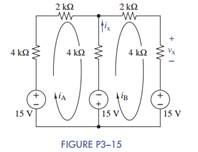Create an Account

Home / Questions / Formulate mesh-current equations for the circuit in Figure P3−15. Arrange the results in...

Formulate mesh-current equations for the circuit in Figure P3−15. Arrange the results in...

(a) Formulate mesh-current equations for the circuit in Figure P3−15. Arrange the results in matrix form Ax = b.

(b) Solve for iA and iB.

(c) Use these results to find vx and ix.Jul 28 2020 View more View LessSubscribe To Get Solution